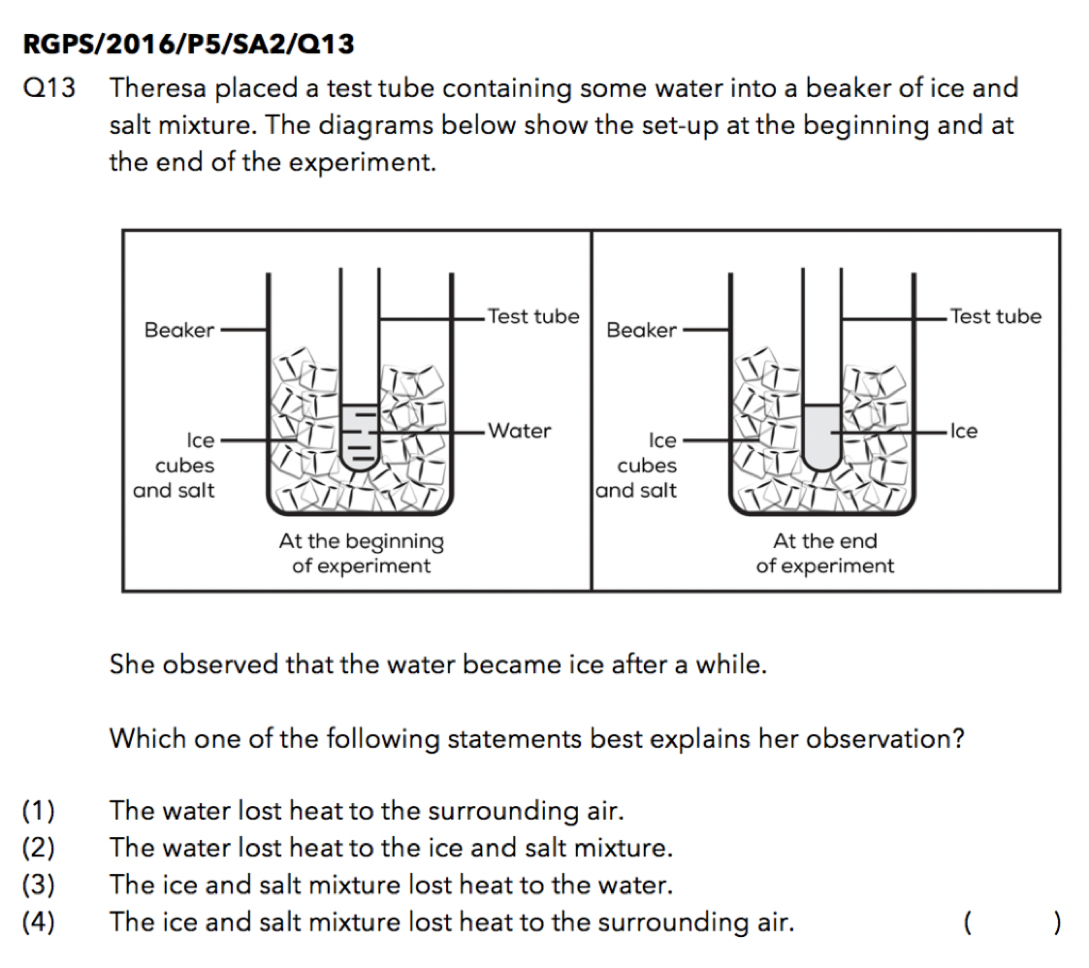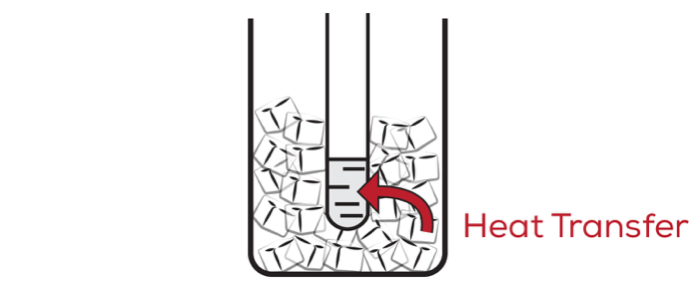Last Updated On

In this article, we will discuss the thought process required to tackle a different application question on Heat that requires students to apply the concept of heat travelling from a hotter to a colder region and the freezing process clearly.

Although most students understand these two concepts, many of them are unable to apply them to application questions accurately. As such, we will be elaborating more on these two concepts and we will share with you how to apply them using an example question.

## QuestionSource: Raffles Girls’ Primary School – 2016 P5 SA2 Science Examination Paper [Q13]

Most students are able to identify that “the water became ice” is the key phrase and recall that water loses heat to freeze and become ice. They then prematurely choose Option (1) The water lost heat to the surrounding air as their answer and move on to the next question, happy that they have managed to solve this question so quickly.

Have you spotted their mistake yet? That’s right, the water will lose heat to the surrounding air! Let us take a look at why and further analyse the four options together.

## Thought Process

Recall the following two concepts that we have learnt in the topic of Heat:

• Heat travels from a hotter region to a colder region.
• Heat gain or loss can result in either a change in temperature of a substance or a change in state of a substance.

In order for the water to freeze into ice, the water must undergo:

• A decrease in temperature – The water must first lose heat to something cooler until it reaches its freezing point, which is 0 ºC.
• Freezing – The water, which is now at 0 ºC, must continue to lose heat until all the water (liquid state) has frozen to become ice (solid state). It should be noted that the temperature of the water remains constant at 0 ºC while it is undergoing the process of freezing.

Thus, in order to explain why the water became ice, we need to choose an option that correctly explains how the temperature of the water decreased to 0 ºC.

## Analysis

### Option (1): The water lost heat to the surrounding air.

This is the option that many students may choose at first glance once they see the phrase “the water lost heat”. However, the water is at the same temperature as the surrounding air, which is definitely not 0 ºC! Once again, recall that heat travels from a hotter to a colder region.

When the two regions have reached the same temperature, there is no heat transfer between the two regions. Since the temperature of the water and the surrounding air is the same, there will not be any heat transfer between these two regions. Therefore, we are unable to say that the water lost heat to the surrounding air. As such, Option (1) is incorrect.

### Option (2): The water lost heat to the ice and salt mixture.

Adding salt lowers the melting point of ice slightly. Although the usual melting point of ice is 0 ºC, adding some salt will cause the melting point of ice to decrease until it is below 0 ºC. In this example, let’s assume that the melting point of ice decreases to -1 ºC after the salt has been added. This means that the ice will start melting at a lower temperature, becoming water at -1 ºC instead of 0 ºC.

SIDE NOTE: Since the melting point of ice is also the freezing point of water, adding salt to water will lower the freezing point of water. This means the water will freeze at a lower temperature, becoming ice at -1 ºC instead of 0 ºC.

Looking at the diagram from the question, we can observe that the ice cubes are still in a solid state at the end of the experiment. Since they have not melted to form water yet, we can infer that the ice cubes are at a temperature below their melting point of -1 ºC.

In order to understand the importance of the ice cubes being at a temperature below -1 ºC, I would like you to consider the following – if the ice cubes were at 0 ºC instead of being below -1 ºC, would the water (liquid state) continue losing heat to freeze and become ice (solid state)?

The answer is no. After the water has lost heat to the ice cubes until it reached 0 ºC, both the water and the ice cubes would be at the same temperature of 0 ºC. As no heat transfer takes place between two regions of the same temperature, the water at 0 ºC would be unable to continue losing heat to the ice cubes at 0 ºC to undergo the freezing process.

Hence, as the ice cubes are at a temperature below -1 ºC, this ensures the following:

• The warmer water in the test tube can lose heat to the cooler ice and salt mixture until it reaches 0 ºC.
• Then, the water at 0 ºC is still warmer than the ice and salt mixture and can continue to lose heat to the cooler ice (which is below -1 ºC) until it freezes to form ice.

Thus, Option (2) correctly explains why the water in the test tube became ice and is the correct answer.

Let us check our understanding of the other two options as well.

### Option (3): The ice and salt mixture lost heat to the water.

This option suggests that heat was transferred from the ice and salt mixture to the water, as we have illustrated in the diagram below:This means that the water gained heat from the ice and salt mixture to increase in temperature. This is incorrect as the water needs to be colder than the ice (recall that heat travels from a hotter region to a colder region) in order for heat to be transferred from the ice to the water. Hence, Option (3) is incorrect.

### Option (4): The ice and salt mixture lost heat to the surrounding air.

Such a scenario would not happen as the ice and salt mixture is cooler than the surrounding air. Instead, the cooler ice and salt mixture should gain heat from the warmer surrounding air. In addition, since the heat transfer described by Option (4) does not involve the water in the test tube, Option (4) is unable to explain why the water froze to become ice. Thus, Option (4) is incorrect.

## Conclusion